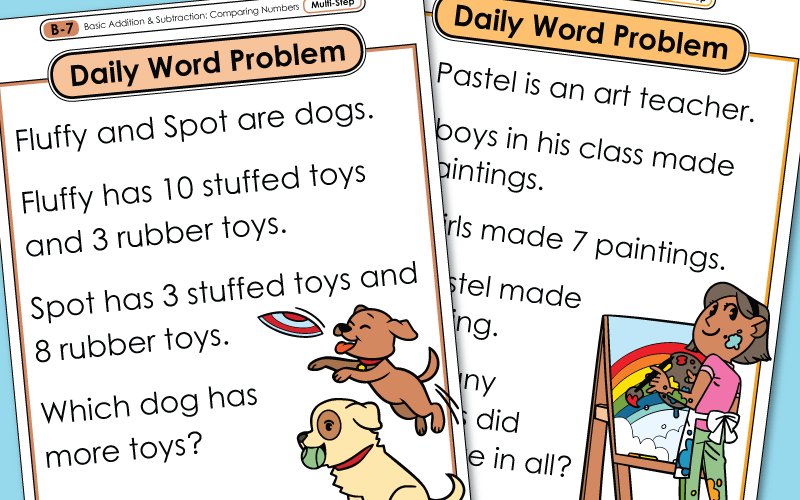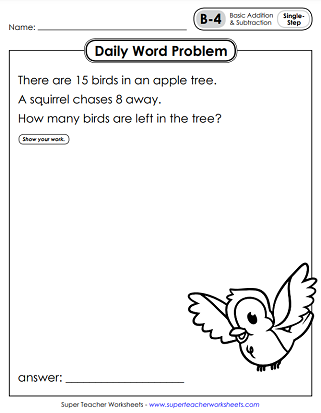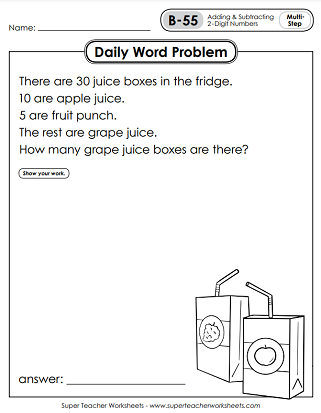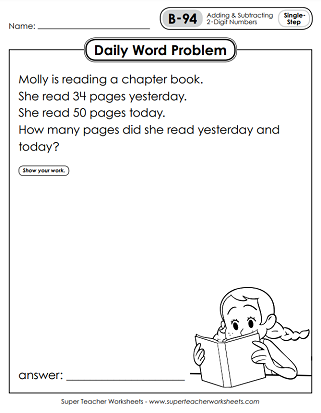# Daily Word Problems - Level B (2nd Grade)

This page contains a collection of Daily Math Word Problem worksheets for 2nd graders. About half are single-step with basic operations; the other half are multi-step. The series starts out by presenting very basic math skills and the problems become more challenging as the year goes on.This file contains 5 worksheets, with one problem on each page. There are 3 questions with multiple-steps and 2 with a single step. (Click the 'Alt' button below if you prefer color poster versions of these problems.)
In these files you have basic addition & subtraction, as well as addition with 3 addends. There's also one on comparing numbers, and repeated addition (multiplication).
Challenge your students to solve these five single and multi-step word problems. Includes multiple addends, comparing, and basic adding & subtracting.
Here are more problems related to basic addition and subtraction, as well as simple repeated addition (multiplication).
On these 2nd grade WPs, kids will find more sums, differences, and products. Half require only one step and half require multiple steps.
This set includes word problems with extra information, basic multiplication (or repeated addition), and multi-step problems involving addition and subtraction.
These five worksheets have a variety of single and multi-step problems involving simple addition with multiple addends and subtraction problems.
These set of daily story problems have questions related to addition, subtraction, and place value.
These worksheets have word problems related to addition, subtraction, and creating arrays.
There are 3 single-step and 2 multi-step problems in this set. Includes sums with multiple addends, as well as reading and interpreting data on a table.
On these worksheets, your children will solve multi-step problems that requires them to add and subtract double-digit numbers.
This set of word problems has 3 multi-step and 2 single-step problems. Skills include basic addition, subtraction, and basic multiplication.
This set of word problems has 1 place value, 2 multi-step, and 2 single-step problems. Skills include basic addition, subtraction, and place value.
This worksheet packet contains math story challenges require students to add and subtract double-digit numbers, compare numbers, multiply (using repeated addition), and skip count.
Here are five more daily word problems, covering a variety of 2nd grade mathematics topics.
Review skills include: reading data on a chart/table; place value; story problems with extra information, multiplication within 5, and odd/even numbers.
This file contains set of worksheets with illustrated 2nd grade math story problems.
Skills on these printables include: odd & even numbers; problems with extra information; addition & subtraction of 2-digit numbers; place value.
One problem describes a secret number. Can your students figure out what it is? Another problem requires children to use repeated addition to solve. There are other problems that require students to find sums and differences of 2-digit numbers.
Calculate the number of fish in a pond using data from a table. Rearrange numbers to find a mystery number. Solve a multi-step problem to calculate how many golf balls a boy has.
This week's daily word problems require skills related to addition, subtraction, telling time, and more!
This week students will interpret a bar graph and practice their addition and subtraction skills.
Math Buzz

Math Buzz is a daily math skills review series for students. Review place value, time, counting money, operations, and much more.

More Daily Word Problems

We have Daily Word Problems for all levels. Have you finished all of the 2nd grade word problems? Jump ahead to 3rd! Looking for something easier? Go back and try the 1st grade set.

## Sample Worksheet ImagesMy Account
Site Information